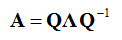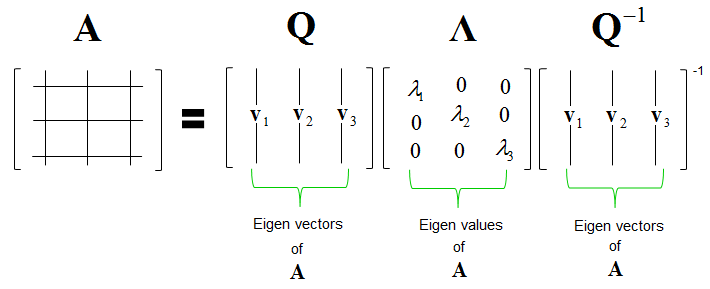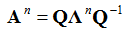Engineering Math - Quick Reference                                 Home : www.sharetechnote.com Eigen Decomposition   Eigen Decomposition is a method of splitting a matrix into multiplication of following three matrix.What does each of the matrix mean ? Each of the matrix in the equation indicates as shown below. Now you would know why this is called Eigen Decomposition.One of the most common application of this decomposition is as follows. You can express a power of matrix as shown below (I wouldn't show you how to prove this. I think you can easily prove this or just use it). In this form, you don't have to do power operation for Q, you only have to power the diagonal matrix which is made up of eigen value. Powering the diagonal matrix is just like powering scalers.